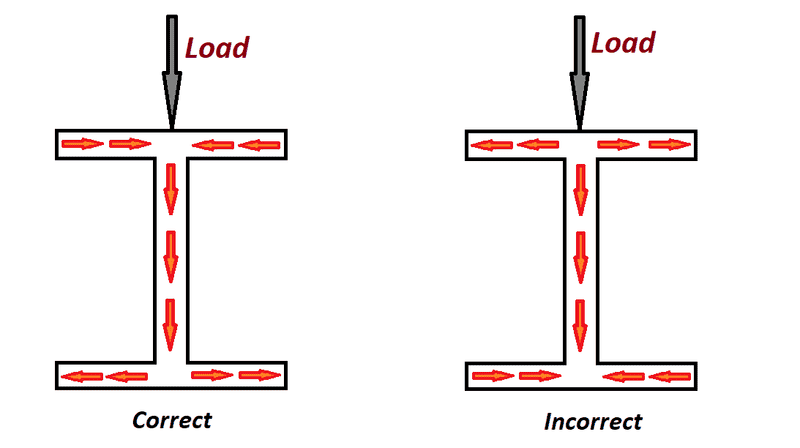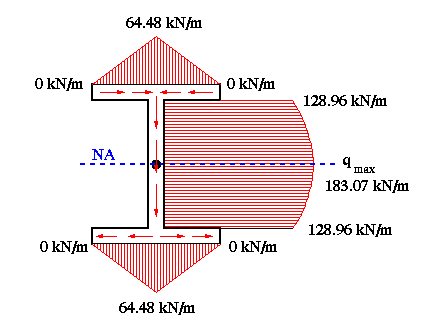# Understanding horizontal shear in beam with vertical load

• davidwinth

#### davidwinth

TL;DR Summary
I am trying to understand both why a horizontal shear is shown in the flange of a beam with a vertical load and how the directions are defined.
In an I-beam, the shear flow is usually shown drawn as on the left, not as drawn on the right. I do not understand why. I do understand:
1. The total horizontal shear force must be zero, consistent with statics.This is satisfied in both images.
2. The vertical direction matches the direction of the external load.
3. There is a longitudinal horizontal shear (in to or out of the plane of the cross section). That, I understand.
My fundamental question is, Why is the image on the left correct and the image on the right incorrect, (or why do we say there is left/right shear at all)? Statics would also be satisfied with no left/right shear.

It matters because, when accounting for the direct shear due to an additional horizontal force (pushing to the right), we will subtract the shears on the upper right hand side of the left I-beam to get the net. If we were to use the right I-beam, we'd have to add the shears to get the net. So the net shear said to exist in the upper right flange of the I-beam depends vitally on the direction assigned to the shear flow, and yet I see no physical reason to favor of one or the other convention.

1. How can I understand why a vertical force produces horizontal (left/right) shear and
2. Why the direction is chosen as it is?Last edited:

Thanks. That is one of the pages I looked at when trying to understand. The problem is that both beams I show are consistent with the "two useful hints" or "guidelines" the author lists. There is no explanation regarding either of my questions.

Thanks. That is one of the pages I looked at when trying to understand. The problem is that both beams I show are consistent with the "two useful hints" or "guidelines" the author lists. There is no explanation regarding either of my questions.
Keep in mind that this shear flow concept was developed for dealing with thin wall cross-sections.
I may be wrong, but this is the way in which I see it:

If you can visualize the flanges of the beam as thin plates, you can see that:
1) The difference of the shear forces in the y-direction are negligible.
2) Is the area of both plates that are connecting to the web the ones "feeling" the pulling and pushing of the axial forces induced by the moments.
3) The shear force next to the edges of a thin plate is close to zero in increase toward the central area of the plate, as the following link shows.

https://en.wikipedia.org/wiki/Bending_of_plates

Therefore, the arrows represent how the flow begins and the free edges of the top flange (just like it starts at the top edge of a rectangular cross-section loaded from the top), increases in magnitude until reaching a max at the neutral line of the beam, to decrease again until reaching the free edges of the bottom flange.
Note that the shear flow for a beam loaded by negative moment would be represented in the opposite direction.•